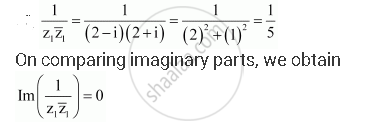CBSE (Arts) Class 11CBSE
Share

# Let Z1 = 2 – I, Z2 = –2 + I. Find Im(1/(Z_1barz_1)) - CBSE (Arts) Class 11 - Mathematics

#### Question

Let z1 = 2 – i, z2 = –2 + i. Find Im(1/(z_1barz_1))

#### SolutionIs there an error in this question or solution?

#### Video TutorialsVIEW ALL 

Solution Let Z1 = 2 – I, Z2 = –2 + I. Find Im(1/(Z_1barz_1)) Concept: Complex Numbers.
S